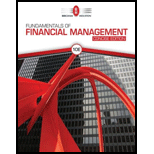Chapter 17, Problem 12P### Fundamentals Of Financial Manageme...

10th Edition
Eugene F. Brigham + 1 other
ISBN: 9781337902571

#### Solutions

Chapter
Section### Fundamentals Of Financial Manageme...

10th Edition
Eugene F. Brigham + 1 other
ISBN: 9781337902571
Textbook Problem

# INTEREST RATE PARITY Assume that interest rate parity holds and that 90-day risk-free securities yield a nominal annual rate of 3% in the United States and a nominal annual rate of 3.5% in the United Kingdom, In the spot market, 1 pound = $1.3. a. What is the 90-day forward rate? b. Is the 90-day forward rate trading at a premium or a discount relative to the spot rate? a) Summary Introduction To determine: The forward rate for 90 days. Interest rate parity refers to that theory, which indicates the difference of interest rates provided by two different countries is to be same as the difference of two types of the exchange rate, which are forward exchange rate and spot exchange rate. Forward exchange rate indicates the pre-decided rate of exchange for currencies of two countries for a date in nearby future. Explanation Given information: The yield of 90 days risk free securities in the Country U is 3% or 0.03. The annual nominal rate in the Country UK is 3.5% or 0.035. The spot market rate is for one pound is$1.3.

Equation of interest rate parity:

Forwad exchange rateSpot exchange rate=(1+rh)(1+rf)

Where,

• rh is yield in Country U dollars.
• rf is yield in pounds.

Compute the forward rate for 90 days:

b)

Summary Introduction

To identify: Whether the 90-day forward exchange rate indicates exchange at premium or discount in comparison to spot rate.

Spot exchange rate indicates the rate for a currency at a present or current time.

### Still sussing out bartleby?

Check out a sample textbook solution.

See a sample solution

#### The Solution to Your Study Problems

Bartleby provides explanations to thousands of textbook problems written by our experts, many with advanced degrees!

Get Started